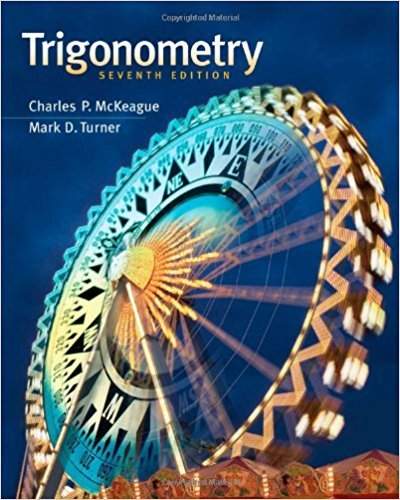×
×

# Solutions for Chapter 8.4: Roots of a Complex Number## Full solutions for Trigonometry | 7th Edition

ISBN: 9781111826857Solutions for Chapter 8.4: Roots of a Complex Number

Solutions for Chapter 8.4
4 5 0 240 Reviews
20
3
##### ISBN: 9781111826857

Chapter 8.4: Roots of a Complex Number includes 60 full step-by-step solutions. Since 60 problems in chapter 8.4: Roots of a Complex Number have been answered, more than 24935 students have viewed full step-by-step solutions from this chapter. This expansive textbook survival guide covers the following chapters and their solutions. Trigonometry was written by and is associated to the ISBN: 9781111826857. This textbook survival guide was created for the textbook: Trigonometry, edition: 7.

Key Math Terms and definitions covered in this textbook
• Affine transformation

Tv = Av + Vo = linear transformation plus shift.

• Cofactor Cij.

Remove row i and column j; multiply the determinant by (-I)i + j •

• Complex conjugate

z = a - ib for any complex number z = a + ib. Then zz = Iz12.

• Diagonalizable matrix A.

Must have n independent eigenvectors (in the columns of S; automatic with n different eigenvalues). Then S-I AS = A = eigenvalue matrix.

• Distributive Law

A(B + C) = AB + AC. Add then multiply, or mUltiply then add.

• Echelon matrix U.

The first nonzero entry (the pivot) in each row comes in a later column than the pivot in the previous row. All zero rows come last.

• Elimination matrix = Elementary matrix Eij.

The identity matrix with an extra -eij in the i, j entry (i #- j). Then Eij A subtracts eij times row j of A from row i.

• Ellipse (or ellipsoid) x T Ax = 1.

A must be positive definite; the axes of the ellipse are eigenvectors of A, with lengths 1/.JI. (For IIx II = 1 the vectors y = Ax lie on the ellipse IIA-1 yll2 = Y T(AAT)-1 Y = 1 displayed by eigshow; axis lengths ad

• Gauss-Jordan method.

Invert A by row operations on [A I] to reach [I A-I].

• Length II x II.

Square root of x T x (Pythagoras in n dimensions).

• Linearly dependent VI, ... , Vn.

A combination other than all Ci = 0 gives L Ci Vi = O.

• Normal equation AT Ax = ATb.

Gives the least squares solution to Ax = b if A has full rank n (independent columns). The equation says that (columns of A)·(b - Ax) = o.

• Nullspace matrix N.

The columns of N are the n - r special solutions to As = O.

• Particular solution x p.

Any solution to Ax = b; often x p has free variables = o.

• Reflection matrix (Householder) Q = I -2uuT.

Unit vector u is reflected to Qu = -u. All x intheplanemirroruTx = o have Qx = x. Notice QT = Q-1 = Q.

• Row space C (AT) = all combinations of rows of A.

Column vectors by convention.

• Spanning set.

Combinations of VI, ... ,Vm fill the space. The columns of A span C (A)!

• Tridiagonal matrix T: tij = 0 if Ii - j I > 1.

T- 1 has rank 1 above and below diagonal.

v + w = (VI + WI, ... , Vn + Wn ) = diagonal of parallelogram.

• Volume of box.

The rows (or the columns) of A generate a box with volume I det(A) I.

×